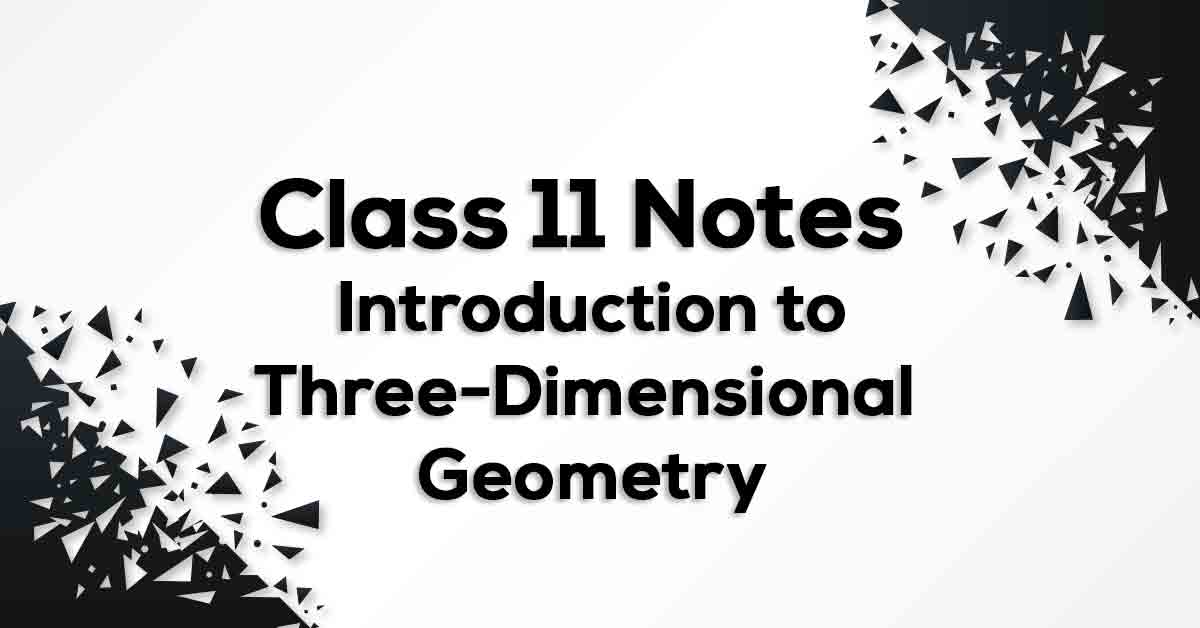Introduction to Three-Dimensional Geometry Class 11 Notes | Vidyakul
×

# Introduction to Three-Dimensional Geometry Class 11 Notes## Class 11 Math Chapter 12 Introduction to Three-Dimensional Geometry Formulae - PDF Download

Introduction to Three-Dimensional Geometry Class 11 Notes are cumulated in a systematic manner which gets rid of confusion among children regarding the course content since CBSE keeps on updating the course every year. The formulae list covers all formulae which provides the students a simple way to study of revise the chapter. Introduction to Three-Dimensional Geometry Class 11 notes would provide you with an effective preparation for your examinations.

### What do you understand by Three-Dimensional Geometry?

To locate position of an object in a line, you just need the distance of the object from the point of reference. To locate the position of a point in a plane, we need two intersecting mutually perpendicular lines in the plane. These lines are called the coordinate axes and the two numbers are called the coordinates of the point with respect to the axes. The point O is called the origin of the coordinate system. The three coordinate planes. These three coordinate planes divide the space into eight parts known as octants.

Know more about the same in Introduction to Three-Dimensional Geometry Class 11 notes pdf.